• 2018-04-11 22:28:14

matplotlib.pyplot.figure 函数内设置，参数名称为figsize。
匹配的关闭函数为matplotlib.pyplot.close
详情参考：https://matplotlib.org/api/_as_gen/matplotlib.pyplot.figure.html#matplotlib.pyplot.figurepython
更多相关内容
• 参考github：...对matplotlib的操作都是在一个figure上完成的，figure是一块画布，类似于现实中的画板。在一块画板上，可以绘制一幅或多幅图形，这些图形就是ax...参考github：

https://github.com/yizhen20133868/Plot-Pictures-Tutorial-for-Paper​github.com

本篇文章是对matplotlib绘图的一些概念、一些通用性函数的综述。

对matplotlib的操作都是在一个figure上完成的，figure是一块画布，类似于现实中的画板。在一块画板上，可以绘制一幅或多幅图形，这些图形就是axes。当只有一个图形时，可以直接使用matplotlib.pyplot（简写为plt）来绘制。

首先我们要引入matplotlib，通用的简写如下：

import matplotlib.pyplot as plt

画图的第一步通常是使用plt.subplots()函数：

生成一块画布。前两个参数为x、y，将画布分成x行y列，每一块是一个axe，返回画布和axes的数组，后面还可以跟一些figure的参数。示例：

fig, axes = plt.subplots(1, 2, figsize = (8, 10))

之后调用各个axe的各个画图函数，即可对每一个小块进行作图。下介绍常用函数。

### 一、通用的一些参数

在调用函数对图形的文字、线条等进行操作时，有些参数是通用的。

• size：设置大小；
• color：设置颜色；
• width：线条、柱体等的宽度

### 二、常用函数

文本设置：

axes.set_xlabel()函数：设置x轴标签。其常用参数有fontdict，设置各个属性。

axes.set_ylabel()函数：设置y轴标签。

axes.set_title()函数：设置标题。

axes.text()函数：添加文本。一般前两个参数为文本的x、y坐标，第三个参数为文本内容。还有两个较常用的参数ha和va，他们都是设置坐标点相对于文本的位置，ha是水平位置，可选的值有'center'、'right'、'left'；va是竖直位置，可选的值有'center'、'top'、'bottom'。完整参数见：https://matplotlib.org/api/_as_gen/matplotlib.pyplot.text.html?highlight=text#matplotlib.pyplot.text

坐标轴、刻度设置：

axes.set_xlim()函数：设置坐标范围。

axes.set_xticks()函数：设置坐标轴刻度。默认情况下是设置的主刻度，如果设置参数minor=True，那么就是设置的分刻度。示例：

axes.set_xticks([1, 2, 3])
axes.set_xticks([0.5, 1.5, 2.5, 3.5], minor=True)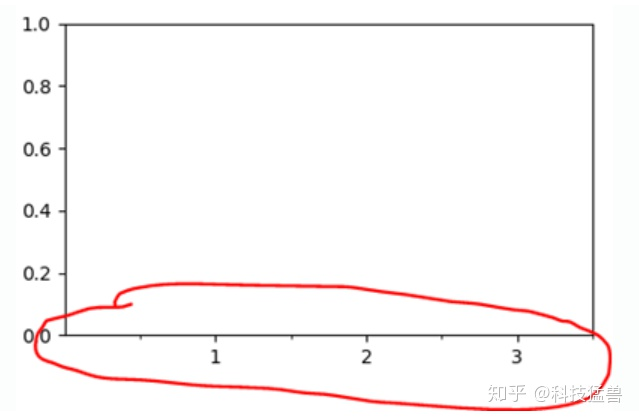axes.set_xticklabels()函数：设置主刻度的标签。

axes.Axis.set_minor_locator()函数：设置坐标轴最小刻度。参数是一个Locator类。示例如下：

axes.yaxis.set_minor_locator(MultipleLocator(0.005))

设置网格线：

axes.grid()函数：画网格线。参数which：表示在哪个刻度画网格线，可选的有‘minor’、‘major’、‘both’。axis：表示在哪个轴画线，可选的有‘x’、‘y’、‘both’。示例：

axe.grid(which = 'minor', c='lightgrey')

图例设置：

axes.legend()函数：设置图例。常用参数为loc，设置位置，可选值有：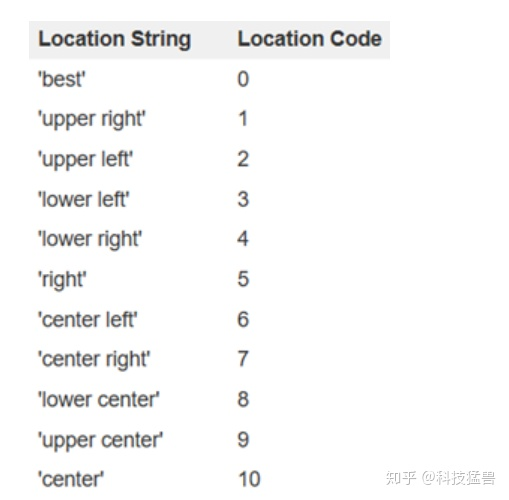完整参数见：https://matplotlib.org/api/legend_api.html?highlight=legend#module-matplotlib.legend

plt.tight_layout()函数可以自动调整子图参数，填充整个图像区域。

### 三、设置字体

设置全局的字体：

plt.rcParams["font.family"] = "Times New Roman"

设置局部的字体，常常改变需要设置的字体的fontdict参数中的family项，示例如下：

plt.title("a", fontdict={'family': 'Times New Roman'})

绘制折线图我们通常使用plot函数画曲线（折线）。每一个plot函数对应一条曲线，画多条线的时候调用多个plot函数即可。

## 四、折线图

plot()函数：

前两个参数为x、y。x：X轴数据，列表或数组；y：Y轴数据，列表或数组。后面还有很多的修饰曲线的参数，常用的有：color控制曲线颜色，linestyle（缩写ls）控制曲线的风格，marker控制数据点的风格等，其对应的常用字符在文章最后的附录。

示例：

fig, axes = plt.subplots(1, 1, figsize=(8, 4))
# 折线图
axes.plot(x, y, linestyle='-', color='#DE6B58', marker='x', linewidth=1.5)
axes.plot(x, y2, linestyle='-', color='#E1A084', marker='x', linewidth=1.5)
# 设置最小刻度间隔
axes.yaxis.set_minor_locator(MultipleLocator(2.5))
axes.xaxis.set_minor_locator(MultipleLocator(0.5))
# 画网格线
axes.grid(which='minor', c='lightgrey')
# 设置x、y轴标签
axes.set_ylabel("Generation Consistency")
axes.set_xlabel("KB Row Number")
# 设置y轴的刻度
axes.set_yticks([70, 75, 80, 85, 90, 95])
# 对每个数据点加标注
for x_, y_ in zip(x, y):
axes.text(x_, y_, y_, ha='left', va='bottom')
for x_, y_ in zip(x, y2):
axes.text(x_, y_, y_, ha='left', va='bottom')
# 展示图片
plt.show()

效果如下：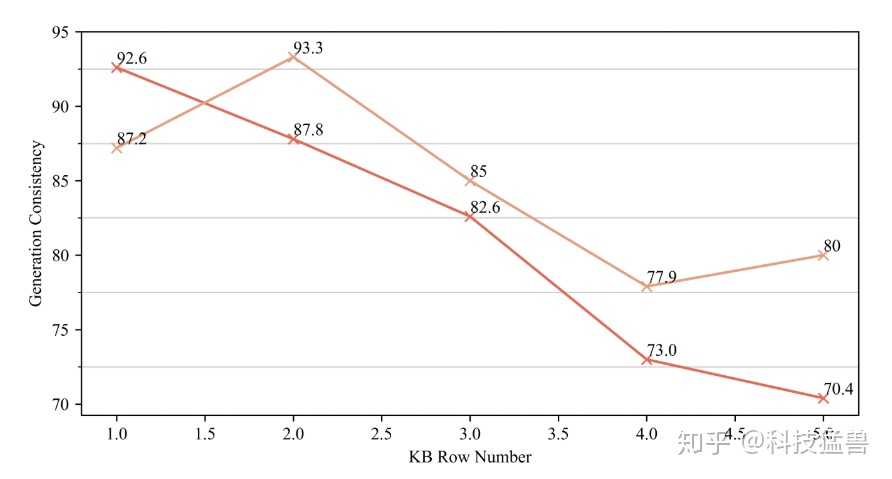## 五、柱状图

### 1、普通柱状图

使用bar函数绘制柱状图，其常用参数如下：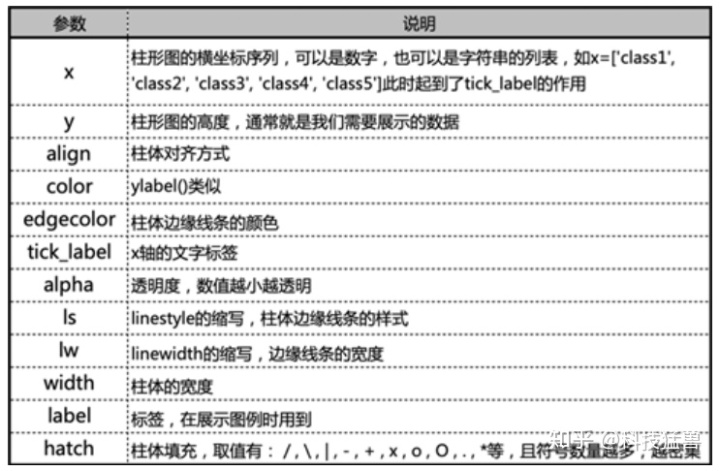其中非常常用的是width、label、alpha、edgecolor、hatch。

此外，常用axe.set_xticks来设置刻度，使用axe.set_xticklabels来设置每个刻度上的标签。

axes.spines['top'].set_visible(False)可以用来设置边框不可见，可选的有top、right、bottom、left四条边。

示例：

fig, axes = plt.subplots(1, 1, figsize=(5, 3))
# 画柱状图
axes.bar(x, a1,  width=0.4, label='height', color="#D2ACA3")
# 图例
axes.legend(loc='best')
# 设置坐标轴刻度、标签
axes.set_xticks([0, 1, 2, 3, 4, 5])
axes.set_yticks([160, 165, 170, 175, 180, 185, 190])
axes.set_ylim((160, 190))
axes.set_xticklabels(['zhouyi', 'xuweijia', 'lurenchi', 'chenxiao', 'weiyu', 'guhaiyao'])
# 设置title
axes.set_title('NLP group members heights')
# 网格线
axes.grid(linewidth=0.5, which="major", axis='y')
# 隐藏上、右边框
axes.spines['top'].set_visible(False)
axes.spines['right'].set_visible(False)

效果：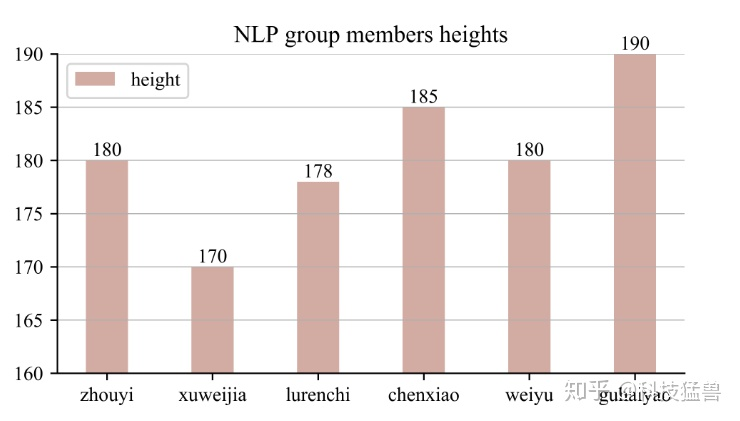### 2、两对柱子竖直方向堆积

底下的柱体和普通柱状图画法一样。

画上面的柱子时，只需要再调用一个bar函数，这个bar函数要添加bottom参数，将bottom的值设置为底下柱子的数值即可。

示例：

axes.bar(x, y1,  width=0.4, label='height', color='#D2ACA3')
axes.bar(x, y2, width=0.4, bottom=y1, color="#EBDFDF", label='increse')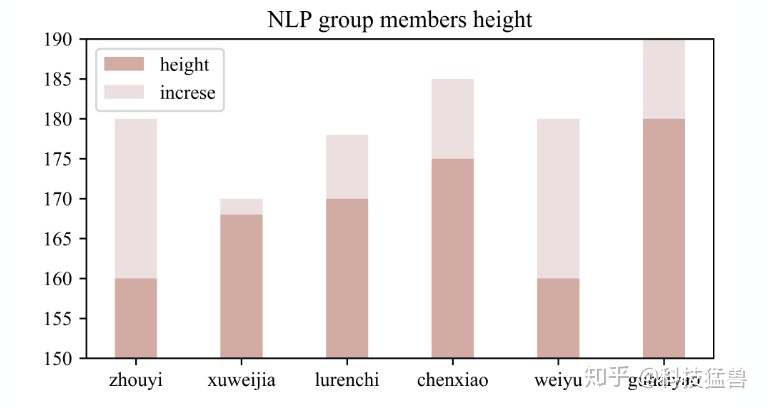### 3、两对柱子并排排列

调用两次bar函数绘制两组柱体。让一组柱子的x值为x-width/2，另一组柱子的x值为x+width/2，即可实现两条柱子并排排列。

示例：

axes.bar(x - width / 2, a1,  width=width, label='2015', color="#D2ACA3")
axes.bar(x + width / 2, a2, width=width, label='2020', color="#EBDFDF")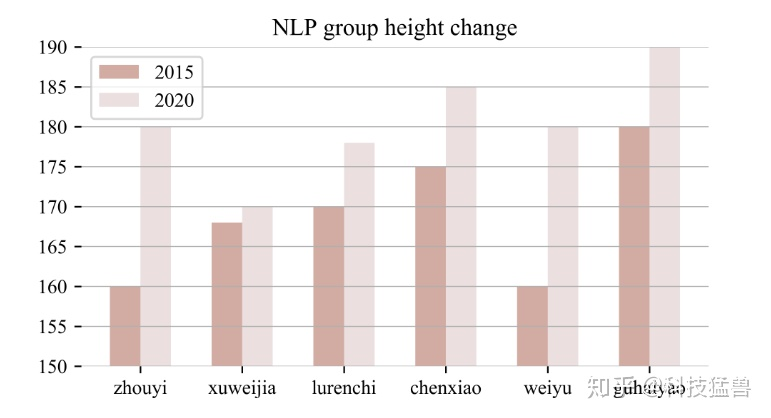### 4、给每根柱体加标注

一般使用循环的形式加text，并将参数ha设置为center、va设置为bottom。用法：

matplotlib.pyplot.text(x, y, s, fontdict=None, withdash=False, **kwargs)
x, y : scalars 防止text的位置。 s : str 内容text。 fontdict : dictionary, optional, default: None 一个定义s格式的dict。

示例：

for i in range(6):
axes.text(x[i], a1[i], a1[i], ha='center', va='bottom')## 三、饼状图

饼图使用plt.pie函数绘制，其各参数如下：其中labels、value、colors、explode是维数相同的列表。还有一些常用的参数：textprops可以设置所有文字（label和value）的属性、wedgeprops可以将饼状图设置为环状图。

pie()函数的返回值为饼片列表、文字标签列表、数值标签列表。可以先获得返回值，再通过setp()函数对相应的文字进行操作、调整。

此外，一般会使用plt.axis('equal')让饼状图看起来更圆。

示例：

fig, axe = plt.subplots(1, 1, figsize=(8, 5))

font1 = {'family': 'Times New Roman', 'size': '15'}
# 饼状图各个部分的标签、值、颜色
labels = ['food', 'clothing', 'housing', 'transport']
values = [0.35, 0.15, 0.2, 0.3]
colors = ['#D2ACA3', '#EBDFDF', '#DE6B58', '#E1A084']
# 突出显示
explode = [0, 0.1, 0, 0]
# 标题
axe.set_title("daily cost", fontdict=font1)
# 画饼状图
wedge, texts, pcts = axe.pie(values, labels=labels, colors=colors, startangle=45, autopct='%3.1f%%'
, explode=explode)
axe.axis('equal')
# 图例
axe.legend(wedge, labels, fontsize=10, title='event', loc=2)
# 设置文本的属性

plt.setp(texts, size=12)
plt.setp(pcts, size=12)
plt.show()

如果给pie函数加上了wedgeprops参数，则可画出环形饼图，示例如下：

将上面的代码中的pie函数的参数增加wedgeprops=dict(width=0.4)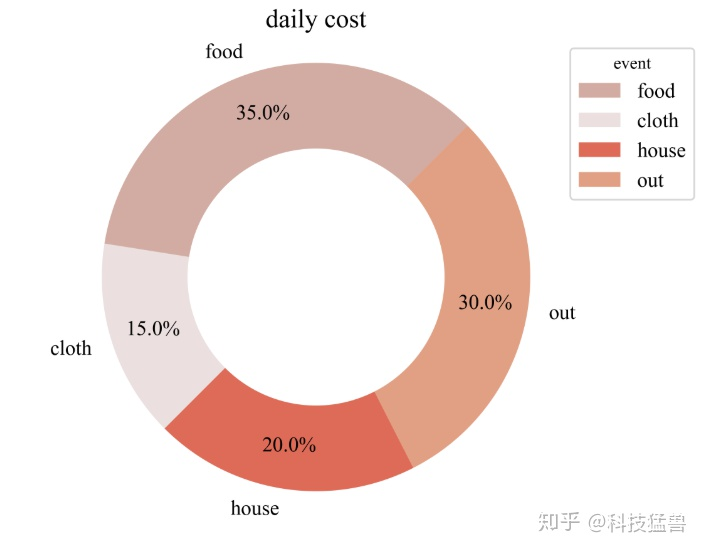展开全文• 设置画布大小 import matplotlib.pyplot as plt fig = plt.figure(figsize=(10,15)) #width=10英寸， height=15英寸 这样就是设置了一个宽度为10英寸，高度为15英寸的画布，注意，英寸！ 但是实际上我们更熟悉的是...

# matplotlib相关

## 设置画布大小

import matplotlib.pyplot as plt
fig = plt.figure(figsize=(10,15)) #width=10英寸， height=15英寸


这样就是设置了一个宽度为10英寸，高度为15英寸的画布，注意，英寸
但是实际上我们更熟悉的是像素值，而不是英寸，那么我们可以这样

fig = plt.figure(figsize=(10,15),dpi=100)	#指定绘图对象的分辨率，即每英寸多少个像素


这样我就指定了一个宽度=100像素高度=150像素的画布。
另外还可以通过

• facecolor: 背景颜色
• edgecolor: 边框颜色
• frameon: 是否显示边框

等属性来做相关设置，具体使用bing搜索figure的属性

fig = plt.figure(figsize=(4,6),dpi=100,facecolor='y',edgecolor='r',frameon=True)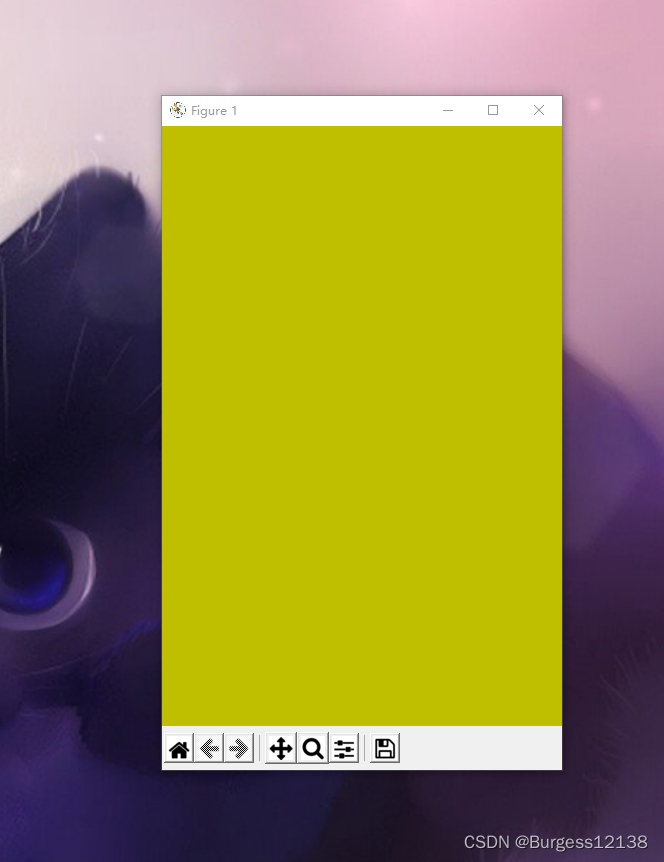## 子图以及坐标轴

示例

import matplotlib.pyplot as plt
fig = plt.figure(figsize=(10,6),dpi=100,facecolor='y',edgecolor='r',frameon=True) #设置一张画布
ax1.spines['right'].set_color('none') #设置1号坐标轴的右框为空
ax2.spines['top'].set_color('r') #设置2号坐标轴的上框为红色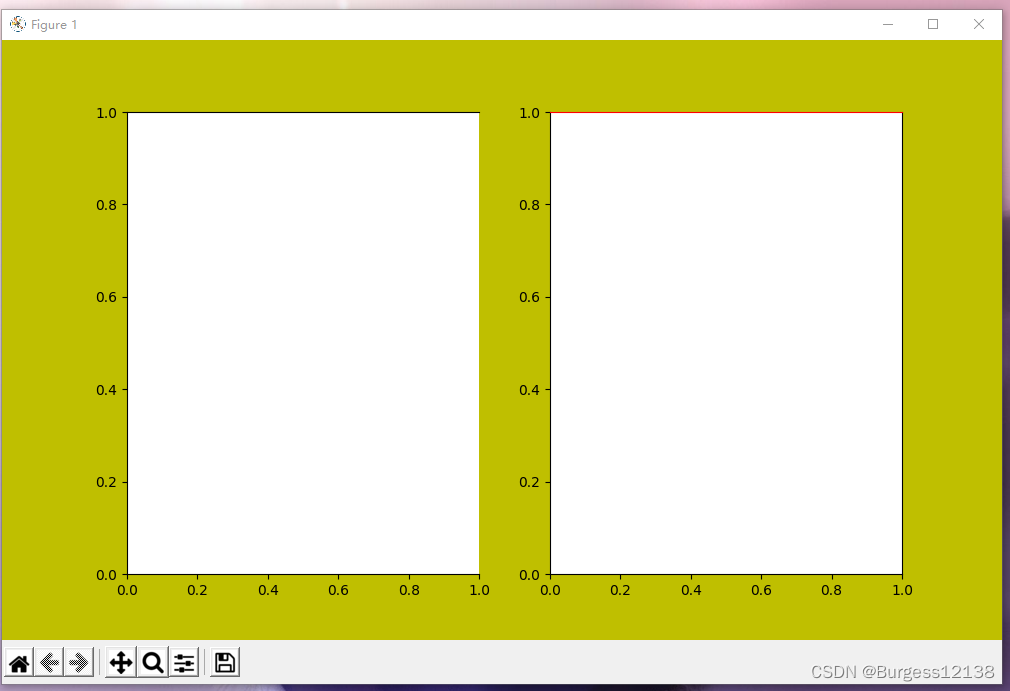ax1.set_xlim(-3,4.5) #设置ax1，即左图的x轴范围是-3到4.5
ax1.set_ylim(-5,7) #同理
ax1.spines['bottom'].set_position(('data',0.8)) #data表示通过值来设置x轴的位置，将x轴绑定在y=0。8的位置
ax1.spines['left'].set_position(('axes',0.5))  #axes表示以百分比的形式设置轴的位置，即将y轴绑定在x轴50%的位置，也就是x轴的中点


以上引自Matplotlib之设置x,y坐标轴的位置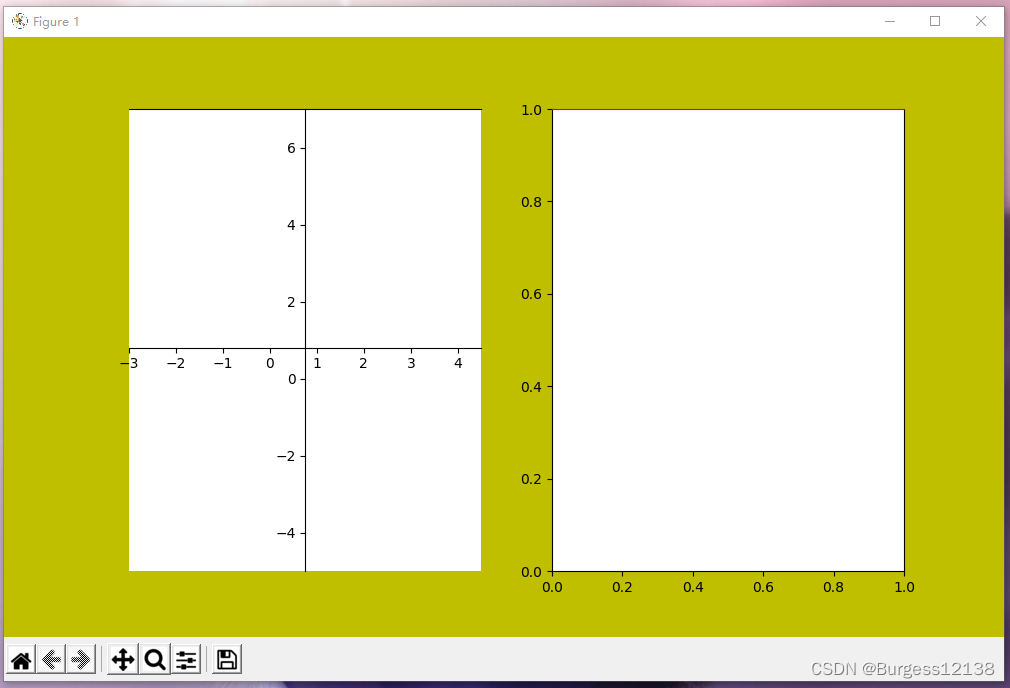ax2.set_ylim(-100,200)
ax2.set_xlim(-150,100)
ax2.spines['left'].set_position(('axes',0.3))
ax2.spines['bottom'].set_position(('axes',0.8))
ax2.spines['bottom'].set_color('r') #设置坐标轴为红色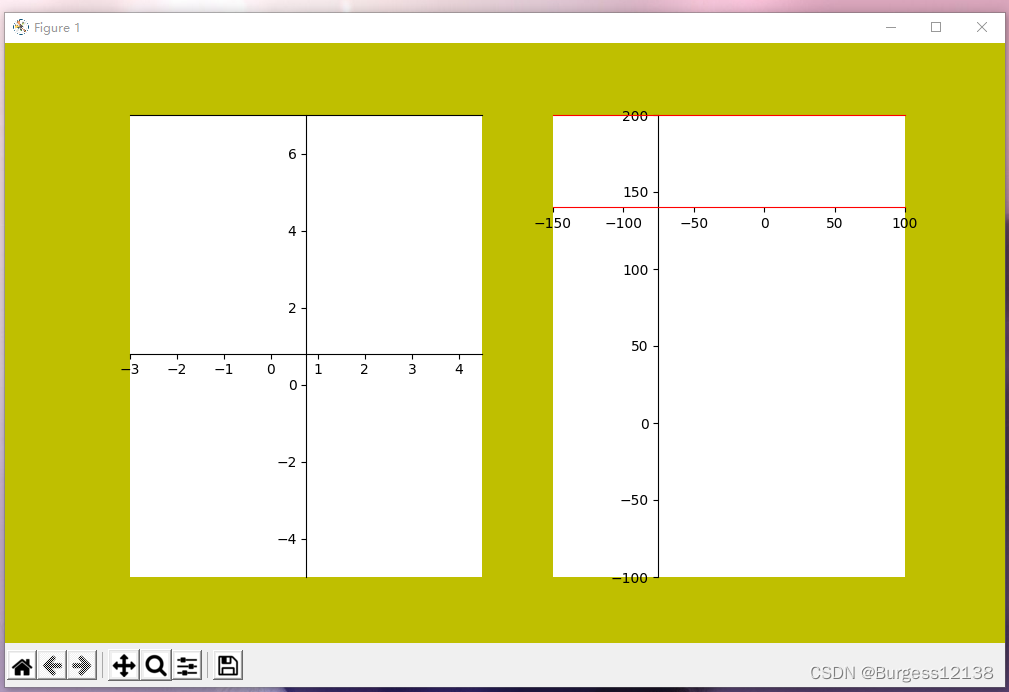ax2.spines['left'].set_visible(False) #右图隐藏纵轴
ax2.xaxis.set_ticks([]) #右图隐藏横轴标签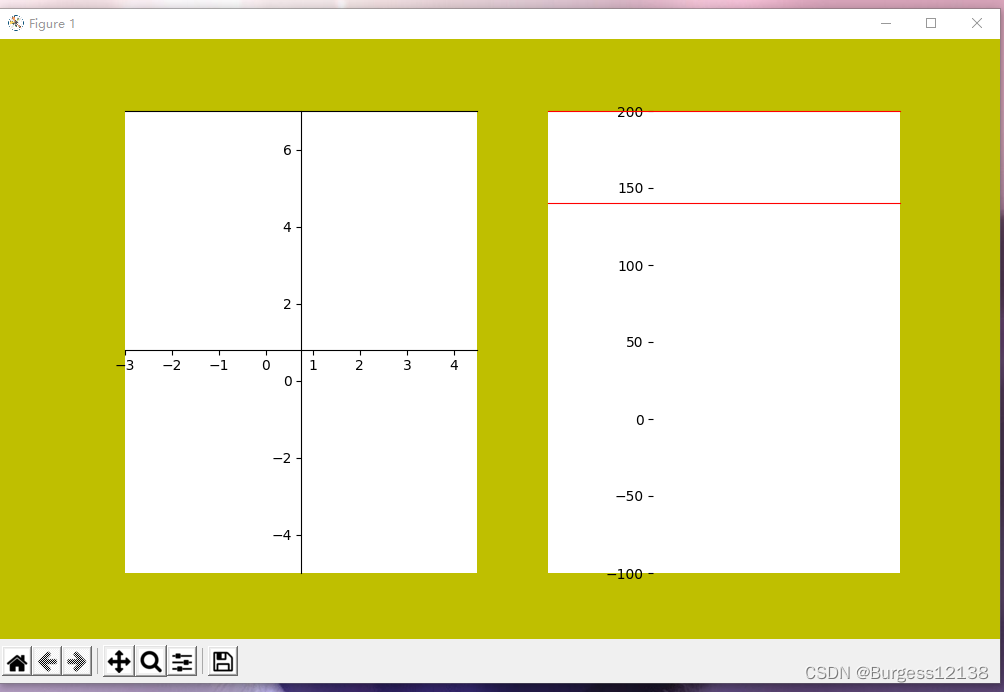删除某一个子图

fig.delaxes(ax2)

展开全文• 只有一个子图时 plt.figure() #默认画布大小 plt.figure( figsize=(width,height) ) #自定义画布大小(width,height) plt.plot(...) #使用plt绘图 有多个子图时（但在一张画布上） 方法1：使用add_subplot # 用 2x2 ...
• 只有一个子图时

plt.figure()                           #默认画布大小
plt.figure( figsize=(width,height) )   #自定义画布大小(width,height)
plt.plot(...)                          #使用plt绘图

• 有多个子图时（但在一张画布上）

# 用 2x2 个子图为例
fig = plt.figure( [figsize=(width,height)] )   #定义整个画布
ax1.plot(...)                                  #在子图上作图
ax2.plot(...)
ax3.plot(...)
ax4.plot(...)

• 方法2：使用subplots
# 仍用 2x2 个子图为例
fig,axes = plt.subplots( 2,2, [figsize=(width,height)] )
ax = axes.flatten()
ax.plot(...)   #若不flatten axes,则这里用axes[0,0]
ax.plot(...)
...


更新的内容

• 调整子图间距

plt.tight_layout()

展开全文python
• import matplotlib.pyplot as plt import numpy （2）、figure对象和subplot简单运用 #figure对象 fig = plt.figure() #figure是图象对象 ax1 = fig.add_subplot(2,2,1) #创建一个2*2的子图，放在第一个位置 ax2 = ...
• 画布的分辨率与大小 import numpy as np import matplotlib.pyplot as plt %matplotlib inline 默认的画布 plt.plot() 设置分辨率 plt.rcParams['figure.dpi'] = 100 plt.plot() 设置图片尺寸 plt.rcParams['...python 大数据
• 这是我对Matplotlib最大的挫折之一。我经常使用光栅数据，例如我想在其中添加彩色地图、图例和一些标题。matplotlib库中的任何简单示例都会导致分辨率不同，因此会重新采样数据。尤其是在做图像分析的时候，你不需要...
• 补充知识：matplotlib 设置图形大小时 figsize 与 dpi 的关系 matplotlib设置图形大小的语句如下： fig = plt.figure(figsize=(a, b), dpi=dpi) 其中： figsize 设置图形的大小，a 为图形的宽， b 为图形的高，...
• matlab设置画布大小代码pyplot教程 代码示例了解用于绘制图形的matplotlib.pyplot接口。 术语 使用指南中的这张图描述了matplotlib图中的所有主要元素： matplotlib 面向对象的Python库进行绘图 图和轴是主要对象 图...
• doc = handout.Handout('Matplotlib教程/Matplotlib常用代码') # 导入绘图模块 import matplotlib.pyplot as plt # 在图表中显示中文字体 from pylab import mpl # 在图表中显示中文字体 # 系统模块，用于自动打开...python
• 这是我对Matplotlib最大的挫折之一.我经常使用栅格数据,例如我想添加色彩图,图例和一些标题.来自matplotlib库的任何简单示例都会导致不同的分辨率,因此重新采样数据.特别是在进行图像分析时,您不需要任何(不需要的)...python画图宽度
• 设置分辨率的时候，画布会改动，配合figsize一起食用更佳。 import matplotlib.pyplot as plt plt.figure(dpi=300,figsize=(2,1) # 分辨率参数-dpi，画布大小参数-figsize
• import matplotlib.pyplot as plt from matplotlib.cbook import get_sample_data from matplotlib.patches import Rectangle imageArray=plt.imread('D:/个人/1.jpg') fig,ax=plt.subplots(1,1) ai=ax.imshow...python
• 如果使用matplotlib.pyplot.imshow默认参数画图的话，结果图像只有一小块 这时候我们把它的aspect调整为auto,就可以自适应大小了 plt.imshow(D, aspect='auto') 结果如下图所示：计算机视觉 opencv 人工智能
• 绘图时设置坐标轴属性 data = np.arange(0,1,0.01) plt.title('my lines example') plt.xlabel('x') plt.ylabel('y') plt.xlim(0,1) plt.ylim(0,1) plt.xticks([0,0.2,0.4,0.6,0.8,1]) plt.yticks([0,0.2,0.4,0.6,...python
• python 更改图形大小Matplotlib中不起作用 - 糯米PHP我在Matplotlib中有一个图，我想通过增加图表的宽度来增加x轴刻度线之间的边距。我想使用这里提到的代码plt.figure(figsize=(20,10)) ->似乎没有任何作用fig...python
• 你也应该会调整单个图的大小了，就是使用如下语句控制单个图形figure的大小，比如我这里设的8*6的。 fig3 = plt.figure(figsize=(8,6)) 但随着继续深入的学习，有时我们很有必要将两个图画在一起，来做对比，所以...python
• Matplotlib实际使用中会有生成不同大小subplots的需求。 import numpy as np import matplotlib.pyplot as plt from matplotlib import gridspec x = np.arange(0, 10, 0.2) y = np.sin(x) fig = plt.figure...
• matplotlib一般使用plt.figure来设置窗口尺寸。 plt.figure(figsize=(16, 9)) 但是如果使用plt.subplots，那么这种方法就无效，只能通过subplots自己设置窗口大小。 fig, subplot_arr = plt.subplots(3,4,figsize=...
• import matplotlib.pyplot as plt # figsize = 11, 9 # figure, ax = plt.subplots(figsize = figsize) x1 =[0,5000,10000, 15000, 20000, 25000, 30000, 35000, 40000, 45000, 50000, 55000] y1=[0, 223, 488, 673...
• Matplotlib基础（一）----创建画布和坐标系 在平时处理数据的时候，因为数据可视化更能显示数据的关系。而python中的matplotlib库很好地提供了我们2D绘图的方式。于是我打算系统且详细的学习matplotlib,并尽可能地...python 开发语言
• 有时我们在使用matplotlib作图时，图片不清晰或者图片大小不是我们想要的，例如下图 上图的相关代码如下： import matplotlib.pyplot as plt x = [10,20,30,40,50,60,70,80,90,1000] y1 = [7,17,27,37,43,49,57,65...python
• Seaborn简介定义Seaborn是一个基于matplotlib且数据结构与pandas统一的统计图制作库。Seaborn框架旨在以数据可视化为中心来挖掘与理解数据。优点代码较少图形美观功能齐全主流模块安装pip命令安装pip install...
• @[TOC](matplotlib matplotlib样式设置、子图操作、图例设置(二))
• Matplotlib中，创建一幅图首先要创建一个画布，但在创建画布前，还需要做一些操作，来使图像能够更好的展示。 # 导入matplotlib库中的pyplot import matplotlib.pyplot as plt # 使图标在丘比特笔记本中展示 %...
• matplotlib一般使用plt.figure来设置窗口尺寸。plt.figure(figsize=(10, 10)) 但是如果使用plt.subplots，那么这种方法就无效，只能通过subplots自己设置窗口大小。fig, ax1 = plt.subplots(figsize=(10, 10)) .....
• 简介使用matplotlib绘图，一个非常基础的问题就是要搞清楚画布和坐标轴。只有真正了解这两个概念，才能获得对整个绘图过程的控制权。画布(Figure)想要绘图就需要一张画布，所有的内容都是放在画布上面的。通过Figure...
• # T-SNE可视化 # 取'Conv1D_1'层 的输出为输出新建为model，采用函数模型 Conv1D_1_layer_model = Model(inputs=model.input, outputs=model.get_layer('Conv1D_1').output) out = Conv1D_1_layer_model.predict(x_...python...